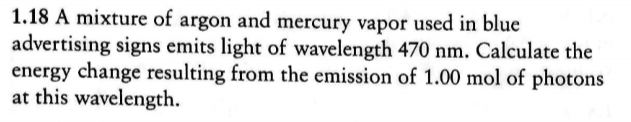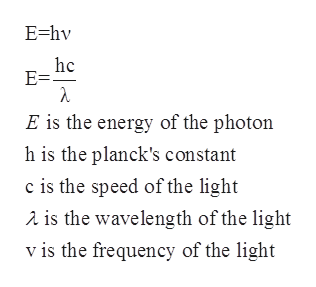# 1.18 A mixture of argon and mercury vapor used in blueadvertising signs emits light of wavelength 470 nm. Calculate theenergy change resulting from the emission of 1.00 mol of photonsat this wavelength

Question
72 viewshelp_outlineImage Transcriptionclose1.18 A mixture of argon and mercury vapor used in blue advertising signs emits light of wavelength 470 nm. Calculate the energy change resulting from the emission of 1.00 mol of photons at this wavelength fullscreen
check_circle

Step 1

The energy ‘E’ which is present in a photon is used to represent the smallest possible ‘packet’ of energy in the electromagnetic wave is directly proportional to the frequency of the wave. Planck’s equation is given as follows:help_outlineImage TranscriptioncloseE-hv hc E = E is the energy of the photon h is the planck's constant c is the speed of the light is the wavelength of the light v is the frequency of the light fullscreen
Step 2

The wavelength is given to be 470 nm =470×10-9 m= 47×10-8 m.

The speed of the light is 3×108 m/s

Planck’s constant = 6.626×10-34 m2kg/s

Substituting all the values in the Planck’s equation as follows:

Step 3

This is the energy of one photon. The energy of one mol of photon can be calcula...

### Want to see the full answer?

See Solution

#### Want to see this answer and more?

Solutions are written by subject experts who are available 24/7. Questions are typically answered within 1 hour.*

See Solution
*Response times may vary by subject and question.
Tagged in
ScienceChemistry

### Physical Chemistry